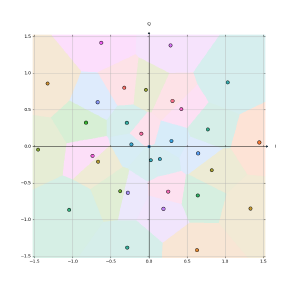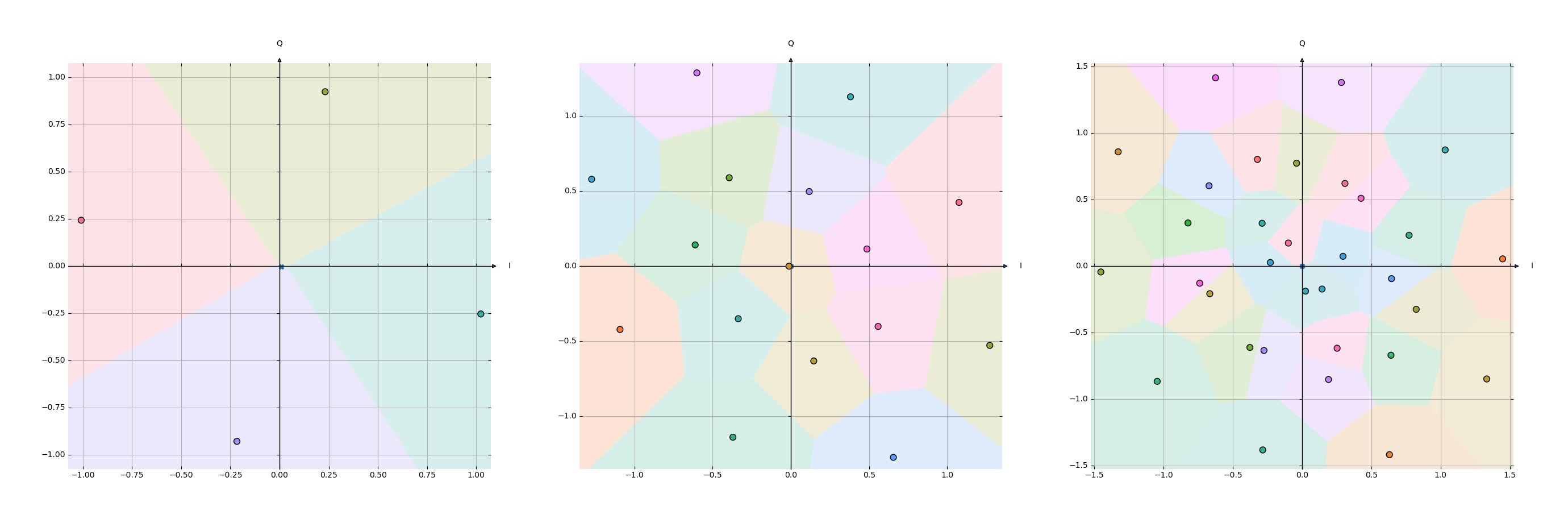matteo
/
constellationnet
Using autoencoders to optimize two-dimensional signal constellations for fiber optic communication systems
You can not select more than 25 topics Topics must start with a letter or number, can include dashes ('-') and can be up to 35 characters long.Mattéo Delabre 73d2572fbe 4 years ago
4 years ago
4 years ago
4 years ago
4 years ago
4 years ago
4 years ago
4 years ago
4 years ago

# ConstellationNet

This is the source code for the “Using Autoencoders to Optimize Two-Dimensional Signal Constellations for Fiber Optic Communication Systems” project.## Structure

The ConstellationNet model is defined inside the `constellation/` folder. At the root are several scripts (described below) for training the model and testing it.

## Available scripts

### Training

`train.py` is a script for training a ConstellationNet network. Hyperparameters are defined at the top of that file and can be changed there. After training, the resulting model is saved as `output/constellation-order-X.pth`, where `X` is the order of the trained constellation.

### Plotting

`plot.py` generates plots of trained models. It loads a trained model from `output/constellation-order-X.pth` where `X` is the order (can be changed at the top of the file). The constellation learned by the encoder is plotted as points and the decision regions learned by the decoder as colored areas around these points.

### Experimentation

`experiment.py` runs experiments to find the best hyperparameters for each constellation order. Currently, the following parameters are tested:

• `order`, the number of points in the constellation, is 4, 16 or 32.
• `initial_learning_rate`, the first learning rate used by the optimizer, is \$10^{-2}\$, \$10^{-1}\$ or \$1\$.
• `batch_size`, the number of training examples in each batch, expressed as a multiple of `order`, is 8 or 2048.
• `first_layer`, the size of the first hidden layer, ranges from 0 to the constellation’s order in steps of 4 (0 meaning that there is no hidden layer).
• `last_layer`, the size of the second hidden layer, ranges from 0 to `first_layer` (because the second layer is never larger than the first).

For a total of 378 different configurations. To enable parallelizing the experimentation, this set of configurations can be partitioned using two arguments, the first one describes the total number of parts to divide the set into and the second one which part number is to be tested.

Results of this experiment are available in `results/experiment.csv`.